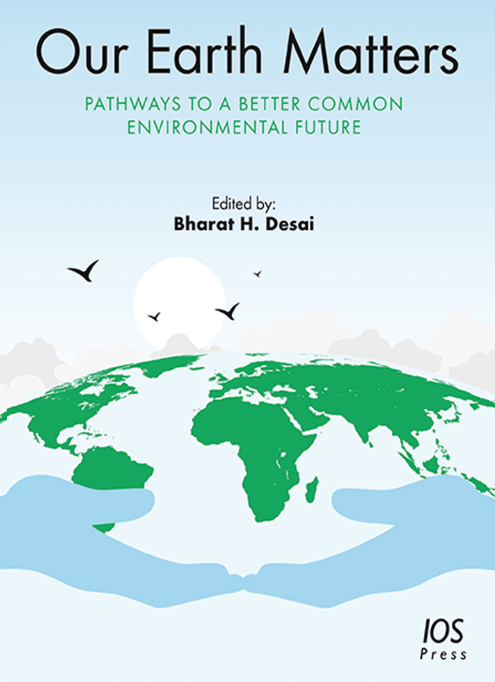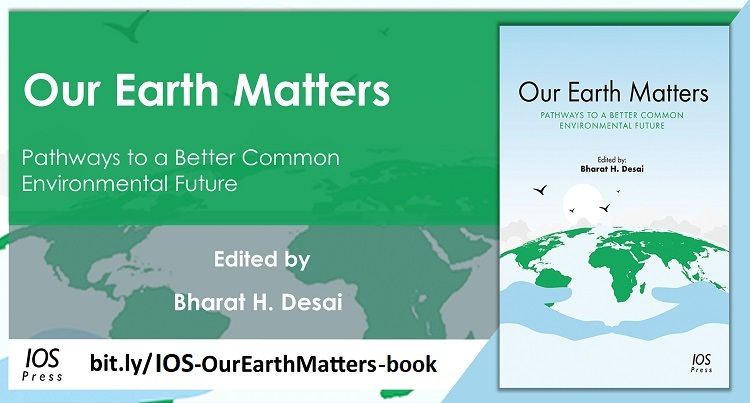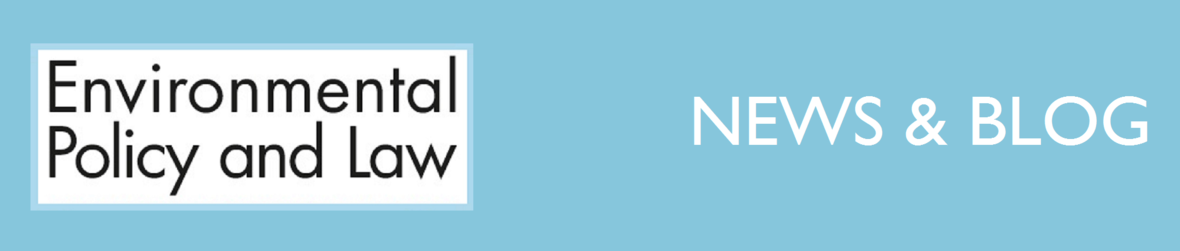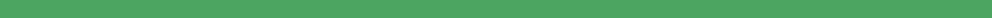Like   Tweet   in
 /* styles */ Please feel free forward to your colleagues and share the news of our new book from EPL

# NEW BOOK: OUR EARTH MATTERS

## Guest Editor: Bharat H. Desai, PhD

 table div table+table+table+table div table{width:100%;padding:0}table div table+table+table+table div table img{width:96.23%;padding:0;float:none}table div table+table+table+table div table td{width:100%;padding:0 1.88% 18px}/* styles */table.module-4{width:99.06%;padding:0}table div table+table+table+table+table div table{width:99.06%;float:none;margin-left:auto;margin-right:auto;padding:0}table div table+table+table+table+table div table a{border:0 none;text-decoration:none}table div table+table+table+table+table div table img{width:100%!important;border:0 none;text-decoration:none}table div table+table+table+table+table div table td{width:100%;padding:0}/* styles */

# Special Offer for our Readers

## Order Your Print Copy at a 20% Discount!*Discounted Print Book Price €97 / US\$119 / £88

As a special offer to our readers and the EPL community, you can now purchase the print book at a 20% discount.

Our Earth Matters, with its 238 pages and 21 outstanding contributions, is currently available for a limited-time only at a discount price. Use the code below when ordering via our website before the end of September and you can get the print book for the discount price: €97 / US\$119 / £88.

Just place your order here before September 30, 2021 and enter the discount code listed below during the ordering process.

## DISCOUNT CODE: EPL20%EARTH

*Discount only applies to the print version; include the eleven digits of the code at checkout

 table div table+table+table+table+table+table+table+table div table{width:100%;padding:0}table div table+table+table+table+table+table+table+table div table img{width:96.23%;padding:0;float:none}table div table+table+table+table+table+table+table+table div table td{width:100%;padding:0 1.88% 18px}/* styles *//* styles */ About the Book Covering a very important theme, Our Earth Matters is an exclusive volume and its content calls for an honest introspection as regards to what we have attained during the past 50 years relating to regulatory processes, and the use of innovative tools and techniques of lawmaking that will take us forward. The primary objective of the book is to raise the awareness of the global audience by inspiring scholars and decision-makers to re-examine current global approaches to environmental issues and explore the future trajectory with new ideas and frameworks for international environmental governance in the 21st century and beyond. The book will be of interest to all those working to secure a sustainable future for planet Earth. View more about the book here & order your print copy with 20% discount here! About the Guest Editor Bharat H. Desai, PhD, is Professor of International Law and Jawaharlal Nehru Chair in International Environmental Law, Centre for International Legal Studies, School of International Studies, Jawaharlal Nehru University, New Delhi; and Editor-in-Chief of Environmental Policy and Law. The book raises pertinent questions about how we might move ahead to forge those pathways to a better environmental future – what have the last 50 years taught us and what are the next steps and priorities? Dr. Desai comments: “Has it brought about changes in human mindsets, jettisoning of greed and defining our needs? What could be the new ideas, approaches, processes, regulatory tools, and institutional structures to address the ‘world problematique’? It still continues to haunt us after 50 years.”table div table+table+table+table+table+table+table+table+table+table+table+table div table{width:100%;padding:0}table div table+table+table+table+table+table+table+table+table+table+table+table div table img{width:96.23%;padding:0;float:none}table div table+table+table+table+table+table+table+table+table+table+table+table div table td{width:100%;padding:0 1.88% 18px}/* styles */## View Related News Posts

Recent Blog Posts

 table div table+table+table+table+table+table+table+table+table+table+table+table+table+table+table div table,table.module-14{width:44.34%;float:left;padding:0}table div table+table+table+table+table+table+table+table+table+table+table+table+table+table+table div table a{border:0 none;text-decoration:none}table div table+table+table+table+table+table+table+table+table+table+table+table+table+table+table div table img{width:100%!important;border:0 none;text-decoration:none}table div table+table+table+table+table+table+table+table+table+table+table+table+table+table+table div table td{width:100%;padding:0 20px 20px 0}/* styles */ World Enivornment Day: Watch the Our Earth Matters Webinar Recording The book was officially launched during our World Environment Day webinar when the contributions were probed and expert scholars discussed these important topics, including the question: what are the steps to take in a move toward more implementation of environmental law? View
 table div table+table+table+table+table+table+table+table+table+table+table+table+table+table+table+table div table,table.module-15{width:44.53%;float:left;padding:0}table div table+table+table+table+table+table+table+table+table+table+table+table+table+table+table+table div table a{border:0 none;text-decoration:none}table div table+table+table+table+table+table+table+table+table+table+table+table+table+table+table+table div table img{width:100%!important;border:0 none;text-decoration:none}table div table+table+table+table+table+table+table+table+table+table+table+table+table+table+table+table div table td{width:100%;padding:0 20px 20px 0}/* styles */ Press Release: Current Global Environmental Law and Policy Are Failing, Experts Say In a landmark special issue of EPL, noted scholars lay out their vision for a complete overhaul of regulatory processes, approaches, and instruments for the protection of the global environment. Contributor Peter Maurer (ICRC) comments: "We all have our part to play as we face this existential threat.” View
 table div table+table+table+table+table+table+table+table+table+table+table+table+table+table+table+table+table div table,table.module-16{width:44.53%;float:left;padding:0}table div table+table+table+table+table+table+table+table+table+table+table+table+table+table+table+table+table div table a{border:0 none;text-decoration:none}table div table+table+table+table+table+table+table+table+table+table+table+table+table+table+table+table+table div table img{width:100%!important;border:0 none;text-decoration:none}table div table+table+table+table+table+table+table+table+table+table+table+table+table+table+table+table+table div table td{width:100%;padding:0 20px 20px 0}/* styles */ Our Earth Matters: Book Published! We are pleased to announce that the limited-edition book Our Earth Matters: Pathways to a Better Common Environmental Future has now been published. It covers regulatory processes and how we will use innovative tools and techniques of lawmaking in the coming years. You can now order your print copy with 20% discount View
 table.module-18{width:99.06%;padding:0}table div table+table+table+table+table+table+table+table+table+table+table+table+table+table+table+table+table+table+table div table{width:99.06%;float:none;margin-left:auto;margin-right:auto;padding:0}table div table+table+table+table+table+table+table+table+table+table+table+table+table+table+table+table+table+table+table div table a{border:0 none;text-decoration:none}table div table+table+table+table+table+table+table+table+table+table+table+table+table+table+table+table+table+table+table div table img{width:100%!important;border:0 none;text-decoration:none}table div table+table+table+table+table+table+table+table+table+table+table+table+table+table+table+table+table+table+table div table td{width:100%;padding:0}/* styles */
 table div table+table+table+table+table+table+table+table+table+table+table+table+table+table+table+table+table+table+table+table div table{width:100%;padding:0}table div table+table+table+table+table+table+table+table+table+table+table+table+table+table+table+table+table+table+table+table div table img{width:96.23%;padding:0;float:none}table div table+table+table+table+table+table+table+table+table+table+table+table+table+table+table+table+table+table+table+table div table td{width:100%;padding:0 1.88% 18px}/* styles */table.module-20{width:99.43%;padding:0}table div table+table+table+table+table+table+table+table+table+table+table+table+table+table+table+table+table+table+table+table+table div table{width:99.43%;float:none;margin-left:auto;margin-right:auto;padding:0}table div table+table+table+table+table+table+table+table+table+table+table+table+table+table+table+table+table+table+table+table+table div table a{border:0 none;text-decoration:none}table div table+table+table+table+table+table+table+table+table+table+table+table+table+table+table+table+table+table+table+table+table div table img{width:100%!important;border:0 none;text-decoration:none}table div table+table+table+table+table+table+table+table+table+table+table+table+table+table+table+table+table+table+table+table+table div table td{width:100%;padding:0}/* styles */table div table+table+table+table+table+table+table+table+table+table+table+table+table+table+table+table+table+table+table+table+table+table+table+table+table+table+table div table{width:100%;padding:0}table div table+table+table+table+table+table+table+table+table+table+table+table+table+table+table+table+table+table+table+table+table+table+table+table+table+table+table div table img{width:96.23%;padding:0;float:none}table div table+table+table+table+table+table+table+table+table+table+table+table+table+table+table+table+table+table+table+table+table+table+table+table+table+table+table div table td{width:100%;padding:0 1.88% 18px}/* styles */